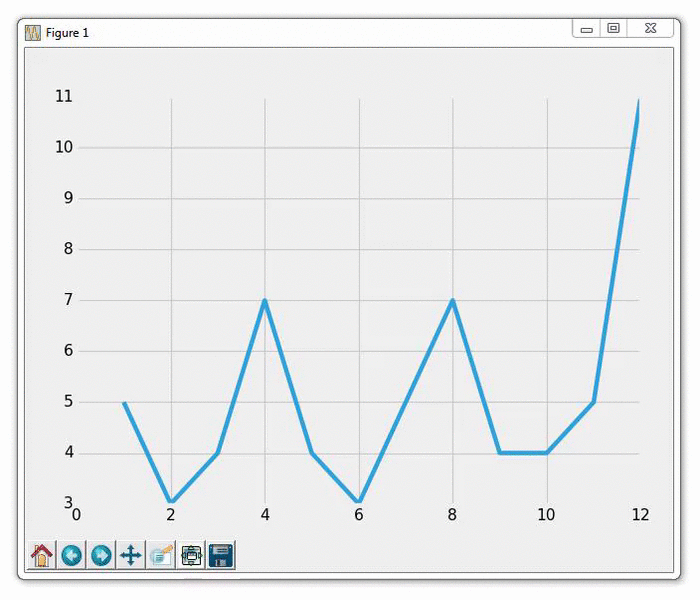## Live Graphs with Matplotlib

In this Matplotlib tutorial, we're going to cover how to create live updating graphs that can update their plots live as the data-source updates. You may want to use this for something like graphing live stock pricing data, or maybe you have a sensor connected to your computer, and you want to display the live sensor data. To do this, we use the animation functionality with Matplotlib.

To start:

```import matplotlib.pyplot as plt
import matplotlib.animation as animation
from matplotlib import style```

Here, the only new import is the matplotlib.animation as animation. This is the module that will allow us to animate the figure after it has been shown.

Next, we'll add some code that you should be familiar with if you're following this series:

```style.use('fivethirtyeight')

fig = plt.figure()

Now we write the animation function:

```def animate(i):
lines = graph_data.split('\n')
xs = []
ys = []
for line in lines:
if len(line) > 1:
x, y = line.split(',')
xs.append(float(x))
ys.append(float(y))
ax1.clear()
ax1.plot(xs, ys)```

What we're doing here is building the data and then plotting it. Note that we do not do plt.show() here. We read data from an example file, which has the contents of:

```1,5
2,3
3,4
4,7
5,4
6,3
7,5
8,7
9,4
10,4```

We open the above file, and then store each line, split by comma, into xs and ys, which we'll plot. Then:

```ani = animation.FuncAnimation(fig, animate, interval=1000)
plt.show()```

We run the animation, putting the animation to the figure (fig), running the animation function of "animate," and then finally we have an interval of 1000, which is 1000 milliseconds, or one second.

The result of running this graph should give you a graph as usual. Then, you should be able to update the example.txt file with new coordinates. Doing this will give you a graph that automatically updates like:The next tutorial:• Introduction to Matplotlib and basic line

• Legends, Titles, and Labels with Matplotlib

• Bar Charts and Histograms with Matplotlib

• Scatter Plots with Matplotlib

• Stack Plots with Matplotlib

• Pie Charts with Matplotlib

• Data from the Internet for Matplotlib

• Converting date stamps for Matplotlib

• Basic customization with Matplotlib

• Unix Time with Matplotlib

• Colors and Fills with Matplotlib

• Spines and Horizontal Lines with Matplotlib

• Candlestick OHLC graphs with Matplotlib

• Styles with Matplotlib

• Live Graphs with Matplotlib
• Annotations and Text with Matplotlib

• Annotating Last Price Stock Chart with Matplotlib

• Subplots with Matplotlib

• Implementing Subplots to our Chart with Matplotlib

• More indicator data with Matplotlib

• Custom fills, pruning, and cleaning with Matplotlib

• Share X Axis, sharex, with Matplotlib

• Multi Y Axis with twinx Matplotlib

• Custom Legends with Matplotlib

• Basemap Geographic Plotting with Matplotlib

• Basemap Customization with Matplotlib

• Plotting Coordinates in Basemap with Matplotlib

• 3D graphs with Matplotlib

• 3D Scatter Plot with Matplotlib

• 3D Bar Chart with Matplotlib

• Conclusion with Matplotlib